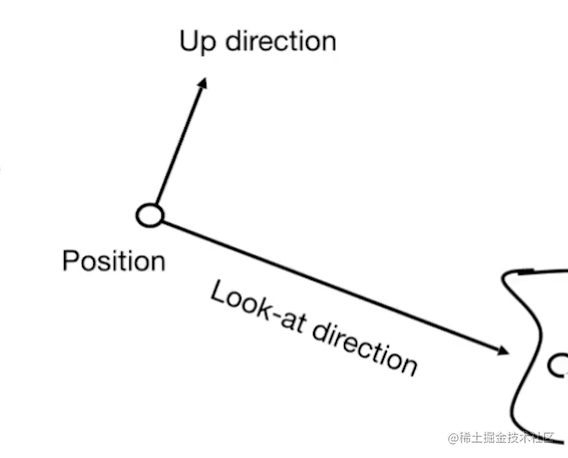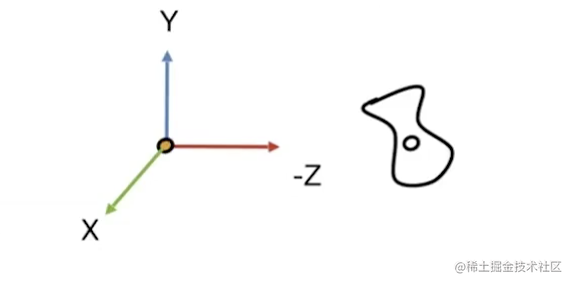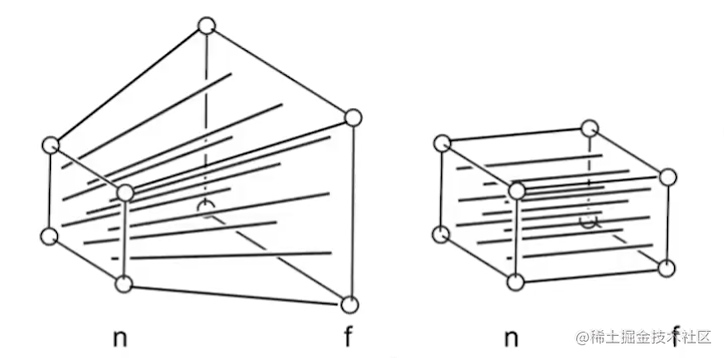# WebGL入门(二)：viewing变换及其数学基础

## 前言

• 一种就是上一篇文章说的modeling变换。
• 还有一种就是接下来说的viewing变换。

Viewing变换也包含两种:

• 视图/摄像机变换
• 投影变换

## Viewing

### 视图变换

• 第一：找一个好地方，把大家或者物体摆好pose（这一步也就是模型变换）
• 第二：找一个好的角度用放置好照相机，并让相机往某个方向看（这一步就是视图变换）
• 最后：“茄子”（这一步就是投影变换）

• 很明显位置是非常重要的，所以第一步就是定义好位置-position(用$\vec e$表示)
• 相机照向哪里也是决定成像的因数，第二步定义相机看向哪里-look-at(用$\vec g$表示)
• 还得定义相机的向上方向，因为如果相机旋转的话，拍出来肯定会有差异，所以定义好向上方向就能把相机固定住(用$\vec t$表示)。• 首先，将位置$\vec e$移动的原点
• 第二，将观察的方向$\vec g$旋转到-z方向上
• 第三，将向上方向$\vec t$旋转到y方向上
• $\vec g$ x $\vec t$方向自然而然的就会旋转到x方向上1、首先确定一下这个逆变换矩阵的形式$R_{view}^{-1}$ = $\begin{bmatrix} {a}&{b}&{c}&{0}\\ {d}&{e}&{f}&{0}\\ {f}&{h}&{i}&{0}\\ {0}&{0}&{0}&{1}\\ \end{bmatrix}$，所以是要求这a-i的这几个变量。

2、第一步将x轴（1,0,0)旋转到$\vec g$ x $\vec t$。在齐次坐标里，x轴向量表示为$\begin{bmatrix} {1}\\ {0}\\ {0}\\ {0}\\ \end{bmatrix}$，最后一位是0。可以求出$R_{view}^{-1}$ = $\begin{bmatrix} {x_{\vec g x \vec t}}&{b}&{c}&{0}\\ {y_{\vec g x \vec t}}&{e}&{f}&{0}\\ {z_{\vec g x \vec t}}&{h}&{i}&{0}\\ {0}&{0}&{0}&{1}\\ \end{bmatrix}$

3、第二步将y轴(0,1,0)旋转到$\vec t$中，可以得出$R_{view}^{-1}$ = $\begin{bmatrix} {x_{\vec g x \vec t}}&{x_{\vec t}}&{c}&{0}\\ {y_{\vec g x \vec t}}&{y_{\vec t}}&{f}&{0}\\ {z_{\vec g x \vec t}}&{z_{\vec t}}&{i}&{0}\\ {0}&{0}&{0}&{1}\\ \end{bmatrix}$

4、第三步将z轴(0,0,1)旋转到-$\vec g$，同理$R_{view}^{-1}$ = $\begin{bmatrix} {x_{\vec g x \vec t}}&{x_{\vec t}}&{x_{\vec {-g}}}&{0}\\ {y_{\vec g x \vec t}}&{y_{\vec t}}&{y_{\vec {-g}}}&{0}\\ {z_{\vec g x \vec t}}&{z_{\vec t}}&{z_{\vec {-g}}}&{0}\\ {0}&{0}&{0}&{1}\\ \end{bmatrix}$

5、旋转矩阵是正交矩阵，所以求逆变换就是其转秩。$R_{view}^{-1}$ -> $R_{view}$，得出$R_{view}$ = $\begin{bmatrix} {x_{\vec g x \vec t}}&{y_{\vec g x \vec t}}&{z_{\vec g x \vec t}}&{0}\\ {x_{\vec t}}&{y_{\vec t}}&{z_{\vec t}}&{0}\\ {x_{\vec {-g}}}&{y_{\vec {-g}}}&{z_{\vec {-g}}}&{0}\\ {0}&{0}&{0}&{1}\\ \end{bmatrix}$

### 投影变换

• 正交投影
• 透视投影

#### 正交投影

• 将摄像机放置到原点，看向-Z方向，向上方向为y轴方向。
• 对比正交投影来说，将物体压扁的操作只需把z轴坐标放弃掉就行
• 然后将所有物体移动和缩放在[-1, 1]的矩形框中。

1、首先将这个立方体的中心点平移到原点。中心点也就是左和右的中心$\frac{r+l}{2}$，上和下的中心$\frac{t+b}{2}$，远和近的中心$\frac{n+f}{2}$。所以能得出$M_t$ = $\begin{bmatrix} {1}&{0}&{0}&{-\frac{r+l}{2}}\\ {0}&{1}&{0}&{-\frac{t+b}{2}}\\ {0}&{0}&{1}&{-\frac{n+f}{2}}\\ {0}&{0}&{0}&{1}\\ \end{bmatrix}$

2、然后通过scale变换将立方体缩放为$[-1, 1]^3$，也就是把这个立方体的边长都变成2，因为[-1,1]。左和右$\frac{2}{r-l}$，上和下$\frac{2}{t-b}$，远和近$\frac{2}{n-f}$。所以是$M_s$ = $\begin{bmatrix} {\frac{2}{r-l}}&{0}&{0}&{0}\\ {0}&{\frac{2}{t-b}}&{0}&{0}\\ {0}&{0}&{\frac{2}{n-f}}&{0}\\ {0}&{0}&{0}&{1}\\ \end{bmatrix}$

3、最后得出$M_ortho = M_s M_t$

### 透视投影

• 首先，将远面通过缩放变换将其变换与近面一样大小的面，将透视投影变为正交投影
• 第二，进行正交投影• 在近面上的所有点经过变换后都不会变换
• 在远面上点中的z经过变换后也不会发生变换

$An + B = n^2\\ Af + B = f^2\\$

$A = n + f\\ B = -nf\\$

webgl专栏